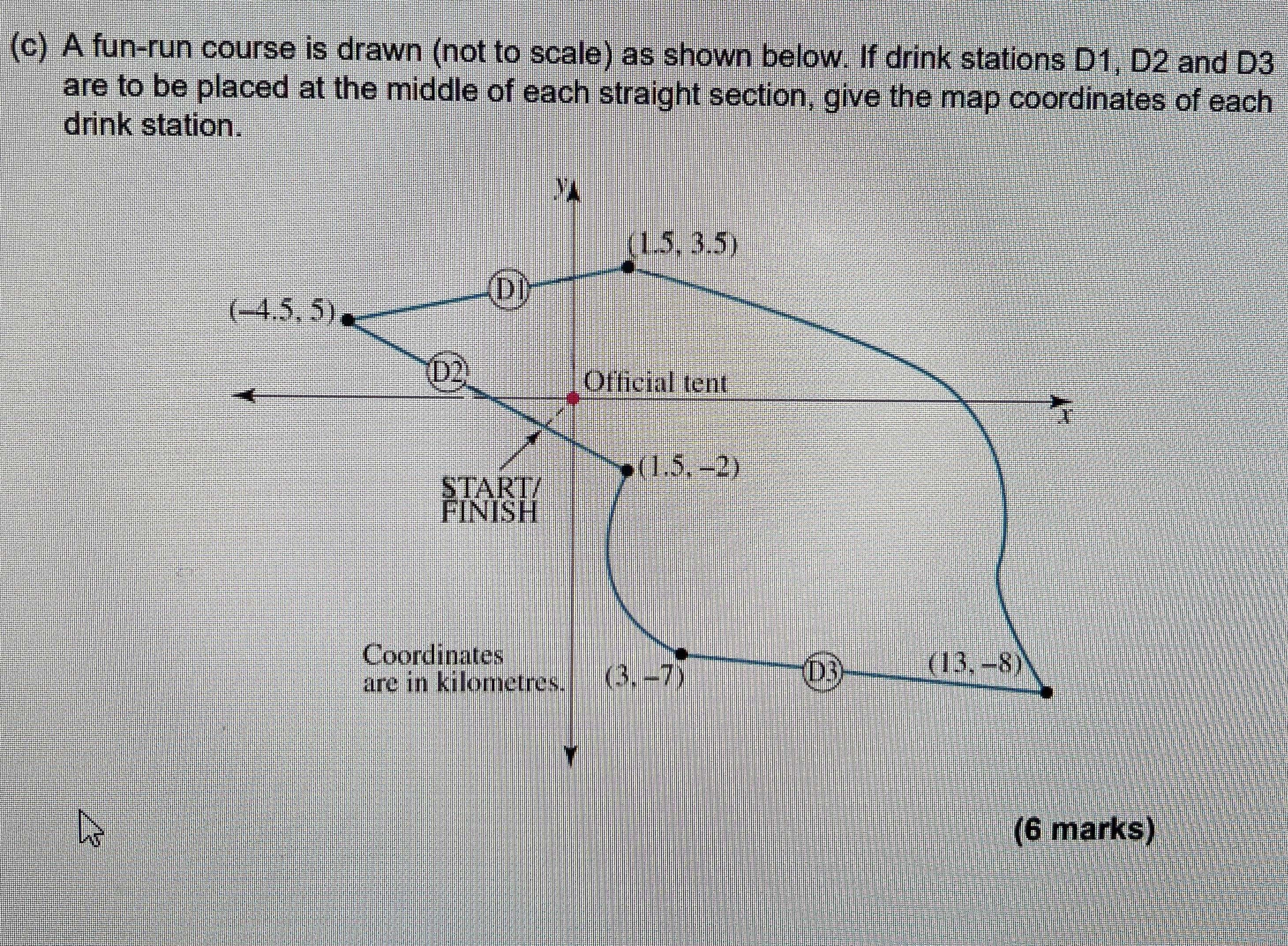### ¿Todavía tienes preguntas de matemáticas?

Pregunte a nuestros tutores expertos
Algebra
PreguntaA fun-run course is drawn (not to scale) as shown below. If drink stations D1, D2 and D3 are to be placed at the middle of each straight section, give the map coordinates of each drink station.

$$D_1 = (- 1.5, 4.25), D_2 = (- 1.5, 1.5), D_3 = (8, - 7.5)$$